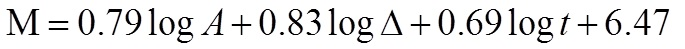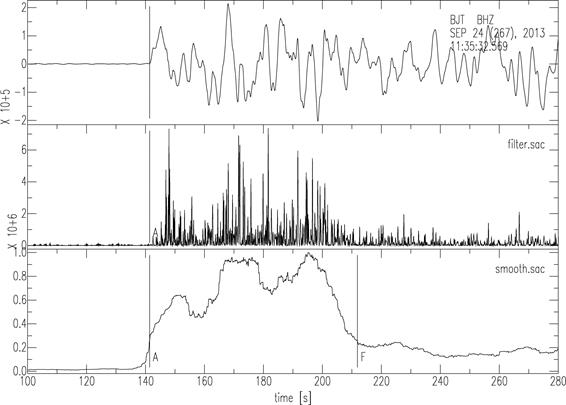## Determination of earthquake magnitudes using duration of high-frequency energy radiation and maximum displacement amplitudes: application to the September 24, 2013 63 km NNE of Awaran, Pakistan earthquake

We applied the magnitude determination method of Hara (2007a, b) to the September 24, 2013 63 km NNE of Awaran, Pakistan earthquake (the origin time: 11:29:47 UTC; the location 26.971üŗN 65.520üŗE depth=15.0km after USGS). In this method, an earthquake magnitude, M, is calculated by the following formula:where A is the maximum displacement (m) during high-frequency energy radiation from the arrival time of a P-wave, Δ is the epicentral distance (km), t is duration (s) of high-frequency energy radiation. The duration of high-frequency energy radiation is estimated by band-pass filtering of first arriving P-waves.
Figure 1 shows an example of measurements of high-frequency energy radiation. The estimated duration is 70.1 sec. The estimated magnitude using the above formula is 7.63, which is consistent with Mww 7.7 from USGS WPhase Moment Tensor Solution, and Mw 7.7 from the Global CMT solution.Fig. 1. An example of measurements of high frequency energy radiation. The upper, middle and lower traces are an observed seismogram, the squares of the band-pass (2-4 Hz) filtered seismogram, and its smoothed time series (normalized by the maximum value), respectively. ügAüh and ügFüh in the lower trace denote the arrival of P-wave and estimated end of high frequency energy radiation, respectively.

Acknowledgements
We retrieved BHZ channel waveform data, recorded at the Global Seismograph Network (GSN) stations, from IRIS DMC (Incorporated Research Institutions for Seismology, Data Management Center). We used SAC (Seismic Analysis Code) (Goldstein et al., 2007) for seismic waveform data analyses.

References

• Goldstein, P., D. Dodge, M. Firpo, L. Minner, J. E. Tull, D. Harris, and W. C. Tapley, SAC - Seismic Analysis Code, http://www.iris.edu/manuals/sac/manual.html, 2007.
• Hara, T., Measurement of duration of high-frequency energy radiation and its application to determination of magnitudes of large shallow earthquakes, Earth Planets Space, 59, 227-231, 2007a.
• Hara, T., Magnitude determination using duration of high frequency energy radiation and displacement amplitude: application to tsunami earthquakes, Earth Planets Space, 59, 561-565, 2007b.

This analysis was done by Tatsuhiko Hara.
Last Updated: 2013/09/26